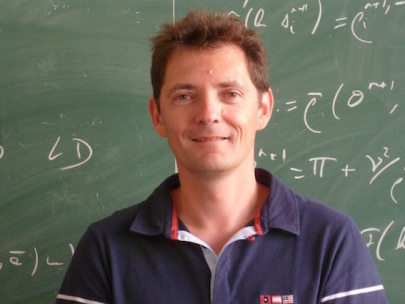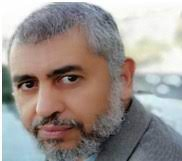Bootstrap Slider

# Invited speakers• K. Ammari ( University of Monastir, Tunisa )
• Title: Regularity and stability of the semigroup associated with some interacting elastic systems: a degenerate damping case
• Abstract : In this talk, we examine regularity and stability issues for two damped abstract elastic systems. The damping involves the average velocity and a fractional power θ, with θ in [−1, 1], of the principal operator. The matrix operator defining the damping mechanism for the coupled system is degenerate. First,we prove that for θ in (1/2, 1], the underlying semigroup is not analytic, but is differentiable for θ in (0, 1); this is in sharp contrast with known results for a single similarly damped elastic system, where the semigroup is analytic for θ in [1/2, 1]; this shows that the degeneracy dominates the dynamics of the interacting systems, preventing analyticity in that range. Next, we show that for θ in (0, 1/2], the semigroup is of certain Gevrey classes. Finally, we show that the semigroup decays exponentially for θ in [0, 1], and polynomially for θ in [−1, 0). To prove our results, we use the frequency domain method, which relies on resolvent estimates. Optimality of our resolvent estimates is also established. Two examples of application are provided.• C. BERTHON ( Nantes University, France )
• Title: Some stability results for second-order finite volume schemes
• Abstract : When approximating weak solutions of hyperbolic systems, the stability of numerical methods is an important and often difficult issue. This stability can be understood in the L1 sense by imposing a robustness argument. Stability in the L2 sense is more difficult to reach and entropy inequalities turn out to be a suitable tool to understand this stability in a weaker sense. Robustness is necessary to guarantee the existence of an approximate solution. This property of robustness is very accessible by first- or second-order numerical methods, eventually by imposing some constraints. Conversely, it is difficult to establish entropy inequalities for first-order numerical schemes and there is no exploitable form for second-order schemes. In this talk, we will show different strategies developed during the last decade to ensure. entropic stability, in a sense to be prescribed, of second-order finite volume schemes.• A. ELMOATAZ ( Caen University, France )
• Title:
• Abstract : .• K. EZZINBI ( Cadi Ayyad University, Morocco)
• Title: Periodic solutions in infinite dimensional dynamical systems: Past and Present
• Abstract : The aim of this work is to present a new method to prove the existence of periodic solutions for dynamical systems well-posed in infinite dimensional spaces. We use the Poincaré map to establish the existence of periodic solutions without compactness of the semigroup generated by the linear part, for that goal we use a new fixed point Theorem that we established recently for affine maps. Several applications are given for evolution equations and partial functional differential equations.• J. FADILI (Caen University, France )
• Title:
• Abstract : .• Y. S. GASIMOV (Azerbaijan University, Azerbaijan)
• Some variable domain eigenvalue problems and applications:
• Abstract : Some eigenvalue problems for the elliptic operators will be considered using the introduced definition for the domain variation. The obtained results will be applied to the investigation of the different problems for the membranes and plates.• A. KHAN (Institute of Technology, Rochester, NY, USA)
• Title: Inverse Problems for Stochastic Variational Equations
• Abstract : This talk will focus on the nonlinear inverse problem of parameter identification in stochastic variational inequalities. We will use the stochastic approximation approach for the inverse problem. Numerical examples will be shown.• L. MANIAR (Cadi Ayyad University, Morocco)
• Title: IDENTIFICATION OF SOURCE TERMS IN HEAT EQUATION WITH DYNAMIC BOUNDARY CONDITIONS
• Abstract : We study an inverse parabolic problem of identifying two source terms in heat equation with dynamic boundary conditions from a final time overdetermination data. Using a weak solution approach by Hasanov, the associated cost functional is analyzed, especially a gradient formula of the functional is proved and given in terms of the solution of an adjoint problem. Next, the existence and uniqueness of a quasi-solution is also investigated. Finally, the numerical reconstruction of some heat sources in a 1-D equation is presented to show the efficiency of the proposed algorithm.• A. Nachaoui (Nantes University, France )
• Title: Cauchy's ptoblem for the modified biharmonic equation: ill-posedness and iterative regularizing methods
• Abstract : The modified biharmonic equation arises naturally when a semi-implicit scheme is used for the discretization of the incompressible Navier–Stokes equations and the resolution of the resulting boundary value problem at each time step is done via the use of the pure stream-function formulation. This equation supplemented with Cauchy boundary conditions gives rise to an inverse Cauchy problem. We show that this problem has a unique solution which does not depend continuously on the given noisy data. We build an iterative regularizing method. We show its convergence and we illustrate its efficiency via several examples.• S. MASNOU ( Claude Bernard Lyon 1 University, France )
• Title: Learning mean curvature flows with neural networks
• Abstract : The mean curvature flow is an emblematic geometric flow which is very naturally related to various numerical and physical applications, e.g. in image / data processing or in material sciences. The talk will be devoted to new, efficient, and accurate numerical methods based on neural networks for the approximation of the mean curvature flow of either oriented or non-orientable surfaces. To learn the correct interface evolution law, the neural networks are trained on phase field representations of exact evolving interfaces. The structure of the networks draws inspiration from splitting schemes used for the discretization of the Allen-Cahn equation. But when the latter approximates the mean curvature motion of oriented interfaces only, the proposed approach extends very naturally to the non-orientable case. In addition, although trained on smooth flows only, the proposed networks can handle singularities as well. Furthermore, they can be coupled easily with additional constraints. Various applications will be shown to illustrate the flexibility and efficiency of our approach: mean curvature flows with volume constraint, multiphase mean curvature flows, numerical approximation of Steiner trees, numerical approximation of minimal surfaces.• J.M. MAZON (Universitat de València, Spain)
• Title:
• Abstract : .

### Technical Secretariat

 ICNTAM2022CONFERENCE Faculté des Sciences et Techniques Sultan Moulay Slimane University Tel : +212 (0) 523 48 51 12/22/82. Fax : +212 (0) 05 23 49 23 39    Campus Mghilla, BP 523 , 23000 Béni Mellal www.icntam.com